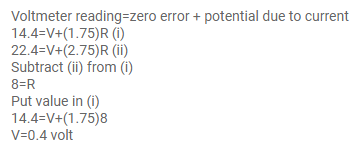# The current in a conductor and the potential difference acrossQuestion:

The current in a conductor and the potential difference across its ends are measured by an ammeter and a voltmeter. The meters draw negligible currents. The ammeter is accurate but the voltmeter has a zero error (that is, it doesn't read zero when no potential difference is applied). Calculate the zero error if the readings for two different conditions are $1.75 \mathrm{~A}, 14.4$ $\mathrm{V}$ and $2.75 \mathrm{~A}, 22.4 \mathrm{~V}$

Solution: